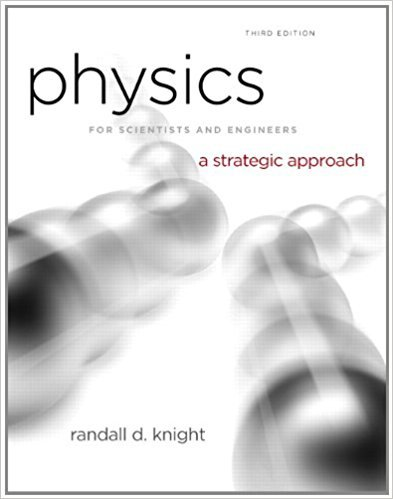×
Get Full Access to Physics For Scientists And Engineers: A Strategic Approach With Modern Physics - 3 Edition - Chapter 12 - Problem 15e
Get Full Access to Physics For Scientists And Engineers: A Strategic Approach With Modern Physics - 3 Edition - Chapter 12 - Problem 15e

×

# The three masses shown in Figure P7.62 are connected byISBN: 9780321740908 69

## Solution for problem 15E Chapter 12

Physics for Scientists and Engineers: A Strategic Approach with Modern Physics | 3rd Edition

• Textbook Solutions
• 2901 Step-by-step solutions solved by professors and subject experts
• Get 24/7 help from StudySoup virtual teaching assistantsPhysics for Scientists and Engineers: A Strategic Approach with Modern Physics | 3rd Edition

4 5 1 308 Reviews
25
4
Problem 15E

Problem 15E

The three masses shown in Figure P7.62 are connected by massless, rigid rods.

a. Find the coordinates of the center of gravity.

b. Find the moment of inertia about an axis that passes through mass A and is perpendicular to the page.

c. Find the moment of inertia about an axis that passes through masses B and C.Step-by-Step Solution:

Solution 15E

Step 1 of 6:

In this question, we need to find coordinates of center of gravity

In part b, we need to find moment of inertia about axis that passes through mass A and is perpendicular to the page

In part c, we need to find moment of inertia about an axis that passes through masses B and C

Step 2 of 6

Step 3 of 6

##### ISBN: 9780321740908

This textbook survival guide was created for the textbook: Physics for Scientists and Engineers: A Strategic Approach with Modern Physics, edition: 3. This full solution covers the following key subjects: Find, moment, passes, axis, inertia. This expansive textbook survival guide covers 17 chapters, and 1439 solutions. Since the solution to 15E from 12 chapter was answered, more than 1873 students have viewed the full step-by-step answer. The answer to “The three masses shown in Figure P7.62 are connected by massless, rigid rods.a. Find the coordinates of the center of gravity.b. Find the moment of inertia about an axis that passes through mass A and is perpendicular to the page.c. Find the moment of inertia about an axis that passes through masses B and C.” is broken down into a number of easy to follow steps, and 55 words. Physics for Scientists and Engineers: A Strategic Approach with Modern Physics was written by and is associated to the ISBN: 9780321740908. The full step-by-step solution to problem: 15E from chapter: 12 was answered by , our top Physics solution expert on 08/30/17, 04:34AM.

Unlock Textbook Solution